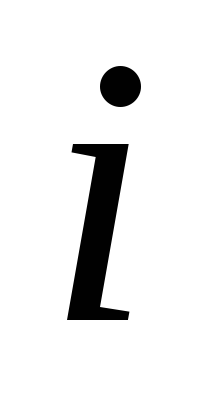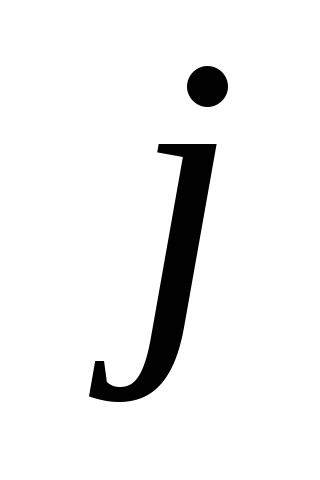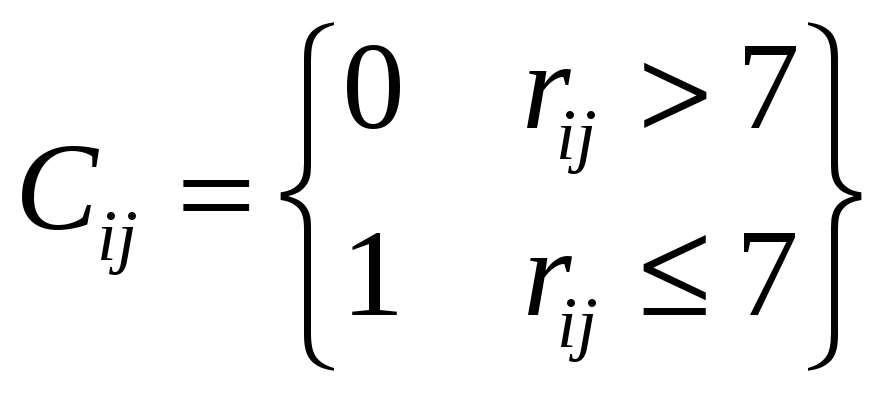# Cs 321: Lecture notes 2 Programs and functions

 for i=1:n coor(i,:) = coor(i,:)-gc; end By clicking on the right top bottom it is possible to rotate the protein chain in 3D. It is not that easy to understand properties of the structure from the 3D view. For example, can you identify how many helices are in the structure? It is useful to have other representations (less straightforward) of the structure that are simpler to analyze. One interesting representation (in 2D) is the contact matrix. If the distance between a pair of amino acidsandis less than seven, the contact matrix is set to one. Otherwise it is set to zeroA short MATLAB script that prepares a contact map is below: n = size(coor,1); for i=1:n cont(i,i) = 0; for j=i+1:n dist = norm(coor(i,:)-coor(j,:)); if (dist<=7) cont(i,j)=1; else cont(i,j)=0; end end end We repeat the same exercise for the protein myoglobin (1mbco). Can you suggest a fingerprint for a helix? Beta sheet? Can you identify sharp turns? Explain the off-diagonal elements and what structural features they correspond to. ---------------------------------------------------------------------------------------------------- Optional Exercise We define the distance between two structures as the norm of the vector differences. The distance between protein structures with coordinate vectors coor1(:,1:3) and coor2(:,1-3) is norm(coor1-coor2). In this exercise we compute the distances between different representations of the same protein. Your report should include the programs, plots and explanations of the results as requested below. Extract the coordinates of the protein 1LAP from the protein data bank Read the CA coordinates of this protein. Plot the chain of the CA-s and the contact matrix. Assign secondary structures. You may also use the plot3 facility. Instead of the CA-s it is possible to read the N atoms. Modify the code to read the N-s instead of the CA-s. Be careful not to lose any N! Stored the coordinates in a different array from the CA array. Compute the distance between the N vector representation of the protein chain and the CA vector of the protein. Repeat the process after placing the geometric centers of the two protein presentations at the origin. Any changes after the shifts of the geometric centers? Download 179.5 Kb.Share with your friends: